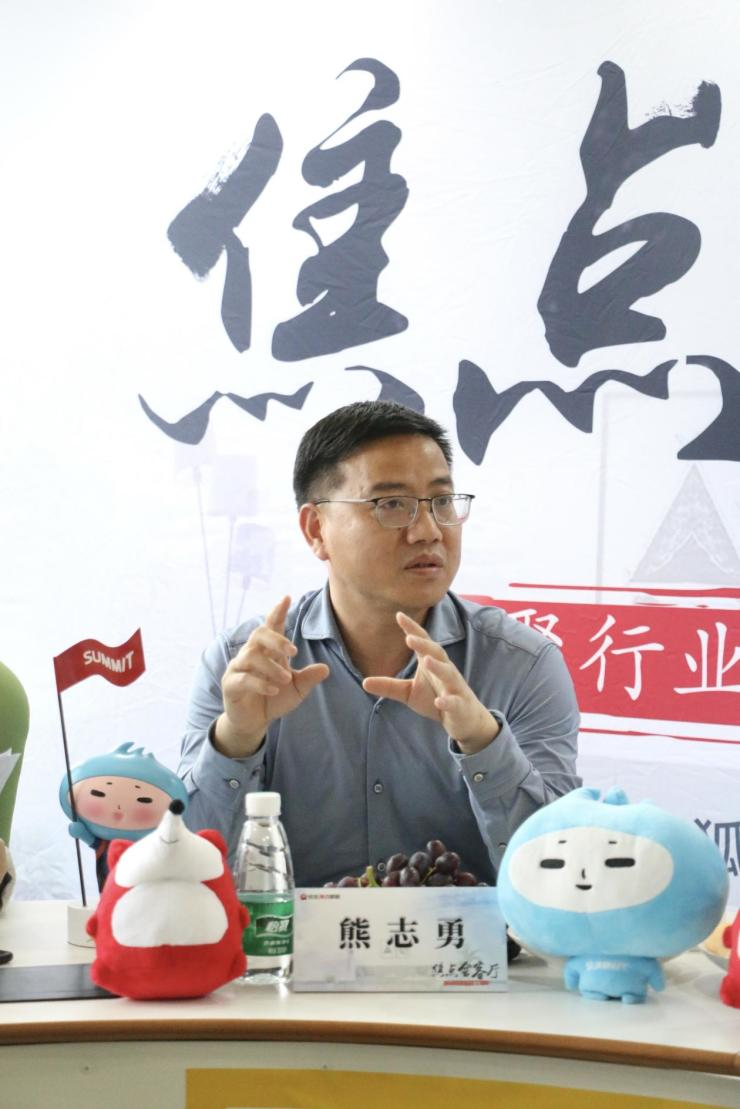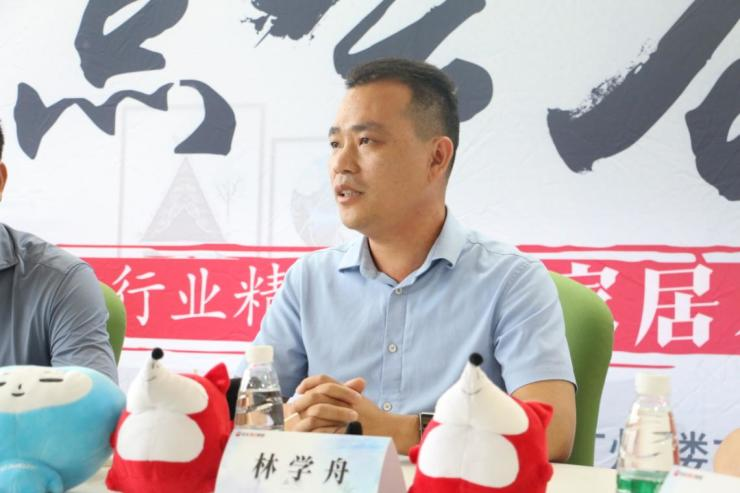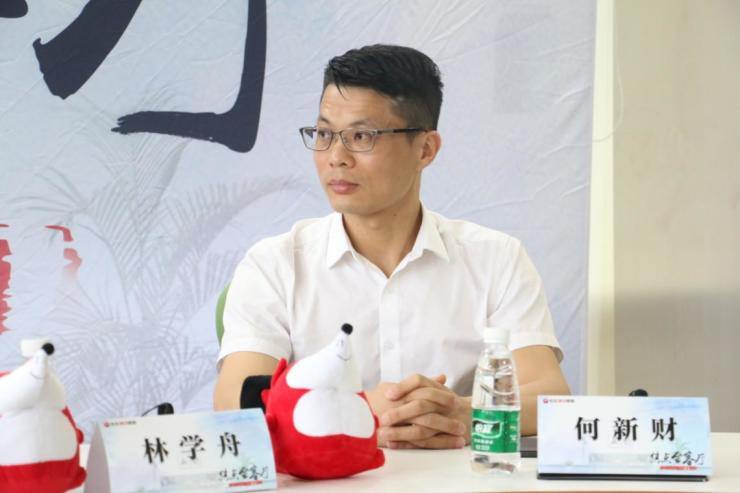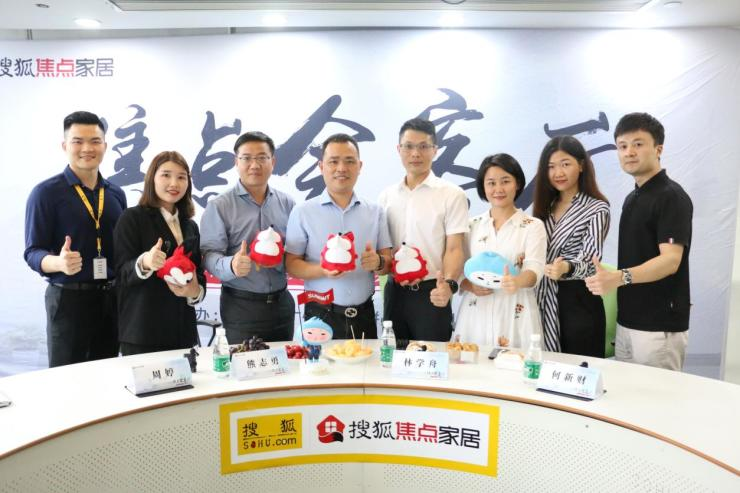|

# 浪鲸x萨米特x必美地板:创新的目的是为消费者解决问题【搜狐焦点家居】：说到创新与变革，最具代表的莫过于商业模式的创新，家居行业的近几年的商业模式创新主要体现在哪些维度？您最看好哪类新模式？

【熊志勇】：家居行业的创新归根到底是为了让客户更省心、通过家居企业提供的产品或者服务让家变得更美，让整个空间变得更舒适、更健康。近两年行业内最明显的创新就是“整装”“大家居定制”开始流行，跨界、联盟、细分、产品链延伸……成为众多家居企业的发展思路与策略，家居企业从单品类产品提供者，向提供一站式服务迈进。萨米特作为陶瓷行业的领军品牌，也一直聚焦消费者的痛点及需求，不断整合资源，提供更优质的服务给消费者。（图/萨米特副总经理 熊志勇）

【林学舟】：首先回顾下家居行业的模式，从厂家的角度来说，一种是厂家直销，一种是经销代理，还有一种是新零售的模式，也就是网销模式。这三种模式大家都在做，目前以第二种居多，第三种属于新模式，大家都在积极探索。新零售的模式就是促使线上线下的互联互通，不仅拉近了厂家与消费者的距离，也让消费者可以在线了解品牌及产品，减少不必要的麻烦

【何新财】：我先介绍下必美地板，成立于1996年的必美国际集团是一家欧洲木业进口投资和市场运营公司，旗下代理世界11大家居品牌，组成欧洲地板一线品牌汇，引领进口地板主流化。这25年以来，必美的核心都在产品。

【搜狐焦点家居】：创新是趋势，但企业也不能为了创新而创新，您认为一个企业进行创新需具备哪些条件？创新的出发点是什么？

【何新财】：我认为创新就是为了解决企业发展过程中遇到的一些问题以及未来可能遇到的问题。以进口地板行业为例，进口地板的发展其实反映了国内消费升级趋势，必美围绕着地板这一品类做到极致，尽可能满足消费者升级的需求。目前必美也在往墙地一体化的模式发展，地板已经不仅仅是地板，也可以是家居板、墙饰板等满足消费者一站式服务的需求。

【林学舟】：浪鲸作为一个从研发、生产到服务、销售为一体的整体卫浴品牌，也经历27年的行业沉淀。我认为创新的前提首先是有长期的战略布局与定位，其次有清晰的目标（图/浪鲸卫浴品牌管理中心总监林学舟）

【熊志勇】：我非常认同林总所说的，企业讲创新一定是站在战略性定位上来讲，偏离企业定位的创新是毫无意义的。创新的必备条件，首先是财力，萨米特陶瓷是一个非常鼓励创新的企业，每年会投入大量的财力做创新的研发投入；其次是人才，创新的主体是人，人才是整个社会最稀缺的资源，企业拥有了人才就拥有了无限可能。萨米特成立博士后研究站、聘请华南理工大学的教授作为顾问，都是重视人才的表现；最后是领导的战略思维高度，自上而下的创新才是最行之有效。我们萨米特瓷砖的叶董事长一直倡导的创新，是基于市场的需求、客户的痛点去做创新，而不是关在实验室里讲创新，这也是萨米特瓷砖能够走在行业前端的关键原因。

【搜狐焦点家居】：创新会带给企业哪些明显的变化？贵司近几年最引以为傲的创新及变革有哪些？可举例说明。

【熊志勇】：刚刚大家都有谈到产品是企业的生命，那我首先说下我们近几年产品方面的创新。萨米特瓷砖的战略目标是打造中国陶瓷行业的国际化品牌，萨米特的定位是年轻化与国际化。所以萨米特也进行了全面的升级，整合全球的科技，不断的自我变革创新。

【林学舟】：刚刚熊总主要讲了产品方面的创新，浪鲸其实在产品方面也有“黑科技”，比如在卫生间的智能化方面，浪鲸重点推出的S12脉霸，它能够实现盖板与陶瓷体一键分离，方便清洁，有利健康。还具有脉冲技术，这些创新都可以带给消费者更好的体验。

【何新财】：前面谈到的核心聚焦点都是产品创新，这一点我也是十分赞同。无论消费模式或者招商渠道如何变化，消费者的需求点始终是好的产品与服务。必美地板每年至少引进欧洲最新产品花色100多款，这些新品可以给设计师的作品增值加分。比如必美比利时宝地雅防水地板，不仅花色时尚，还可以做到24小时防水；而必美意大利费列德罗，在整个地板行业都是顶尖产品，是最高端的纯手工制造的地板，深受欧洲贵族喜爱，也非常适合我国的精英阶层。总之，必美地板会通过不同的产品，去匹配不同消费群体的需求。（图/必美国际集团市场品牌总监何新财）

【搜狐焦点家居】：跨界是家居行业中经常看到的创新形式，近两年家居企业跨界出圈事件频发，并多次成为行业话题，例如定制家居品牌转型整装，红星美凯龙布局智能家居生产等，您如何看待这类事件？

【熊志勇】：我认为跨界也好升级也罢，都是基于市场和客户需求而做的事情，是顺势而为。萨米特瓷砖如果不升级做健康瓷砖，如何打造全球化品牌。企业跨界的初衷也是为了更好地解决客户遇到的问题，给消费者提供更好的服务，同时也让企业发展的更好，让整个行业发展的更好。

【林学舟】：跨界确实比较火，我们在去年也做了一些跨界破圈的动作，比如联合慕思V6、大自然地板等一起直播带货，各大品牌共用消费者资源，这是营销方面的跨界合作；还有一些跨品类甚至跨行业的大跨界，比如许多人开始布局整装及装配式装修。之所以会有这些跨界的动作，一方面是国家政策的倡导，装配式装修确实是未来的方向，另一方面也是消费者的需求推动。浪鲸卫浴也开始从卫生间跨到其他空间，立足产品，不断提升品牌的综合竞争力。目前整装的流行，我认为是好事，这对企业来说也是一种改变自我的方式，也有利于节能减排，对整个社会的未来发展也是好事。【何新财】：所有的跨界合作，都是最大化的满足消费者装修的需求，消费者需要的不仅仅是单个品类，他要的是一个家，而通过品牌跨界整合营销，可以最大化、最快速的解决消费者的材料需求

【搜狐焦点家居】：五一黄金周即将来临，往年许多品牌往往以促销为主，形式都大同小异，消费者其实已经没有太大感觉，今年贵司将如何通过五一节日营销创新，打造出差异化呢？

【何新财】：家居行业一直以来是以促销为主，但我认为促销不应该是单纯的让利或者返点，家居的消费者第一立足点是在于买到自己喜欢的好的产品。今年五一，必美会更注重品牌价值感输出，通过品牌价值塑造，更好的销售，让消费者真真切切感受到必美的产品工艺完全区别于其他普通地板，价值感的塑造远比降价促销重要。。今年五一，必美会更注重品牌价值感输出，通过品牌价值塑造，更好的销售，让消费者真真切切感受到必美的产品工艺完全区别于其他普通地板，价值感的塑造远比降价促销重要。

【林学舟】：对于浪鲸卫浴来说，每个月都是黄金周，我们现在的促销也已经不是简单的降价之类的。比如我们刚刚结束的一场落地活动，就是联合台球冠军潘晓婷进行全年的营销赋能。通过明星效应加持，与当地优秀设计师一起推动浪鲸卫浴在当地的知名度与影响力。其次是品牌IP塑造，浪鲸每年都有品牌日活动IP，在全国抽取100名客户去巴厘岛旅行，真正回馈消费者。

【熊志勇】：萨米特这次也主要是围绕品牌增值宣传，会趁节日做一些真正让利消费者的活动，比如天猫商城的消费抵用券。整个5月，我们更多的是围绕“登山节”IP去营销，希望萨米特能够带给消费者积极正面，健康的品牌印象【搜狐焦点家居】： 非常感谢今天三位嘉宾的精彩分享，创新是企业生存发展的根本，也是一个企业的核心竞争力。企业任何创新与变革的最终目标都是“用户”。现代管理大师彼得·德鲁克说：为客户创造价值是企业的唯一使命。

`声明：本文由入驻焦点开放平台的作者撰写，除焦点官方账号外，观点仅代表作者本人，不代表焦点立场错误信息举报电话： 400-099-0099，邮箱：jubao@vip.sohu.com，或点此进行意见反馈，或点此进行举报投诉。`

A B C D E F G H J K L M N P Q R S T W X Y Z
A - B - C - D - E
• A
• 鞍山
• 安庆
• 安阳
• 安顺
• 安康
• 澳门
• B
• 北京
• 保定
• 包头
• 巴彦淖尔
• 本溪
• 蚌埠
• 亳州
• 滨州
• 北海
• 百色
• 巴中
• 毕节
• 保山
• 宝鸡
• 白银
• 巴州
• C
• 承德
• 沧州
• 长治
• 赤峰
• 朝阳
• 长春
• 常州
• 滁州
• 池州
• 长沙
• 常德
• 郴州
• 潮州
• 崇左
• 重庆
• 成都
• 楚雄
• 昌都
• 慈溪
• 常熟
• D
• 大同
• 大连
• 丹东
• 大庆
• 东营
• 德州
• 东莞
• 德阳
• 达州
• 大理
• 德宏
• 定西
• 儋州
• 东平
• E
• 鄂尔多斯
• 鄂州
• 恩施
F - G - H - I - J
• F
• 抚顺
• 阜新
• 阜阳
• 福州
• 抚州
• 佛山
• 防城港
• G
• 赣州
• 广州
• 桂林
• 贵港
• 广元
• 广安
• 贵阳
• 固原
• H
• 邯郸
• 衡水
• 呼和浩特
• 呼伦贝尔
• 葫芦岛
• 哈尔滨
• 黑河
• 淮安
• 杭州
• 湖州
• 合肥
• 淮南
• 淮北
• 黄山
• 菏泽
• 鹤壁
• 黄石
• 黄冈
• 衡阳
• 怀化
• 惠州
• 河源
• 贺州
• 河池
• 海口
• 红河
• 汉中
• 海东
• 怀来
• I
• J
• 晋中
• 锦州
• 吉林
• 鸡西
• 佳木斯
• 嘉兴
• 金华
• 景德镇
• 九江
• 吉安
• 济南
• 济宁
• 焦作
• 荆门
• 荆州
• 江门
• 揭阳
• 金昌
• 酒泉
• 嘉峪关
K - L - M - N - P
• K
• 开封
• 昆明
• 昆山
• L
• 廊坊
• 临汾
• 辽阳
• 连云港
• 丽水
• 六安
• 龙岩
• 莱芜
• 临沂
• 聊城
• 洛阳
• 漯河
• 娄底
• 柳州
• 来宾
• 泸州
• 乐山
• 六盘水
• 丽江
• 临沧
• 拉萨
• 林芝
• 兰州
• 陇南
• M
• 牡丹江
• 马鞍山
• 茂名
• 梅州
• 绵阳
• 眉山
• N
• 南京
• 南通
• 宁波
• 南平
• 宁德
• 南昌
• 南阳
• 南宁
• 内江
• 南充
• P
• 盘锦
• 莆田
• 平顶山
• 濮阳
• 攀枝花
• 普洱
• 平凉
Q - R - S - T - W
• Q
• 秦皇岛
• 齐齐哈尔
• 衢州
• 泉州
• 青岛
• 清远
• 钦州
• 黔南
• 曲靖
• 庆阳
• R
• 日照
• 日喀则
• S
• 石家庄
• 沈阳
• 双鸭山
• 绥化
• 上海
• 苏州
• 宿迁
• 绍兴
• 宿州
• 三明
• 上饶
• 三门峡
• 商丘
• 十堰
• 随州
• 邵阳
• 韶关
• 深圳
• 汕头
• 汕尾
• 三亚
• 三沙
• 遂宁
• 山南
• 商洛
• 石嘴山
• T
• 天津
• 唐山
• 太原
• 通辽
• 铁岭
• 泰州
• 台州
• 铜陵
• 泰安
• 铜仁
• 铜川
• 天水
• 天门
• W
• 乌海
• 乌兰察布
• 无锡
• 温州
• 芜湖
• 潍坊
• 威海
• 武汉
• 梧州
• 渭南
• 武威
• 吴忠
• 乌鲁木齐
X - Y - Z
• X
• 邢台
• 徐州
• 宣城
• 厦门
• 新乡
• 许昌
• 信阳
• 襄阳
• 孝感
• 咸宁
• 湘潭
• 湘西
• 西双版纳
• 西安
• 咸阳
• 西宁
• 仙桃
• 西昌
• Y
• 运城
• 营口
• 盐城
• 扬州
• 鹰潭
• 宜春
• 烟台
• 宜昌
• 岳阳
• 益阳
• 永州
• 阳江
• 云浮
• 玉林
• 宜宾
• 雅安
• 玉溪
• 延安
• 榆林
• 银川
• Z
• 张家口
• 镇江
• 舟山
• 漳州
• 淄博
• 枣庄
• 郑州
• 周口
• 驻马店
• 株洲
• 张家界
• 珠海
• 湛江
• 肇庆
• 中山
• 自贡
• 资阳
• 遵义
• 昭通
• 张掖
• 中卫

1室1厅1厨1卫1阳台

1
2
3
4
5

0
1
2

1

1

0
1
2
3报名成功，资料已提交审核A B C D E F G H J K L M N P Q R S T W X Y Z
A - B - C - D - E
• A
• 鞍山
• 安庆
• 安阳
• 安顺
• 安康
• 澳门
• B
• 北京
• 保定
• 包头
• 巴彦淖尔
• 本溪
• 蚌埠
• 亳州
• 滨州
• 北海
• 百色
• 巴中
• 毕节
• 保山
• 宝鸡
• 白银
• 巴州
• C
• 承德
• 沧州
• 长治
• 赤峰
• 朝阳
• 长春
• 常州
• 滁州
• 池州
• 长沙
• 常德
• 郴州
• 潮州
• 崇左
• 重庆
• 成都
• 楚雄
• 昌都
• 慈溪
• 常熟
• D
• 大同
• 大连
• 丹东
• 大庆
• 东营
• 德州
• 东莞
• 德阳
• 达州
• 大理
• 德宏
• 定西
• 儋州
• 东平
• E
• 鄂尔多斯
• 鄂州
• 恩施
F - G - H - I - J
• F
• 抚顺
• 阜新
• 阜阳
• 福州
• 抚州
• 佛山
• 防城港
• G
• 赣州
• 广州
• 桂林
• 贵港
• 广元
• 广安
• 贵阳
• 固原
• H
• 邯郸
• 衡水
• 呼和浩特
• 呼伦贝尔
• 葫芦岛
• 哈尔滨
• 黑河
• 淮安
• 杭州
• 湖州
• 合肥
• 淮南
• 淮北
• 黄山
• 菏泽
• 鹤壁
• 黄石
• 黄冈
• 衡阳
• 怀化
• 惠州
• 河源
• 贺州
• 河池
• 海口
• 红河
• 汉中
• 海东
• 怀来
• I
• J
• 晋中
• 锦州
• 吉林
• 鸡西
• 佳木斯
• 嘉兴
• 金华
• 景德镇
• 九江
• 吉安
• 济南
• 济宁
• 焦作
• 荆门
• 荆州
• 江门
• 揭阳
• 金昌
• 酒泉
• 嘉峪关
K - L - M - N - P
• K
• 开封
• 昆明
• 昆山
• L
• 廊坊
• 临汾
• 辽阳
• 连云港
• 丽水
• 六安
• 龙岩
• 莱芜
• 临沂
• 聊城
• 洛阳
• 漯河
• 娄底
• 柳州
• 来宾
• 泸州
• 乐山
• 六盘水
• 丽江
• 临沧
• 拉萨
• 林芝
• 兰州
• 陇南
• M
• 牡丹江
• 马鞍山
• 茂名
• 梅州
• 绵阳
• 眉山
• N
• 南京
• 南通
• 宁波
• 南平
• 宁德
• 南昌
• 南阳
• 南宁
• 内江
• 南充
• P
• 盘锦
• 莆田
• 平顶山
• 濮阳
• 攀枝花
• 普洱
• 平凉
Q - R - S - T - W
• Q
• 秦皇岛
• 齐齐哈尔
• 衢州
• 泉州
• 青岛
• 清远
• 钦州
• 黔南
• 曲靖
• 庆阳
• R
• 日照
• 日喀则
• S
• 石家庄
• 沈阳
• 双鸭山
• 绥化
• 上海
• 苏州
• 宿迁
• 绍兴
• 宿州
• 三明
• 上饶
• 三门峡
• 商丘
• 十堰
• 随州
• 邵阳
• 韶关
• 深圳
• 汕头
• 汕尾
• 三亚
• 三沙
• 遂宁
• 山南
• 商洛
• 石嘴山
• T
• 天津
• 唐山
• 太原
• 通辽
• 铁岭
• 泰州
• 台州
• 铜陵
• 泰安
• 铜仁
• 铜川
• 天水
• 天门
• W
• 乌海
• 乌兰察布
• 无锡
• 温州
• 芜湖
• 潍坊
• 威海
• 武汉
• 梧州
• 渭南
• 武威
• 吴忠
• 乌鲁木齐
X - Y - Z
• X
• 邢台
• 徐州
• 宣城
• 厦门
• 新乡
• 许昌
• 信阳
• 襄阳
• 孝感
• 咸宁
• 湘潭
• 湘西
• 西双版纳
• 西安
• 咸阳
• 西宁
• 仙桃
• 西昌
• Y
• 运城
• 营口
• 盐城
• 扬州
• 鹰潭
• 宜春
• 烟台
• 宜昌
• 岳阳
• 益阳
• 永州
• 阳江
• 云浮
• 玉林
• 宜宾
• 雅安
• 玉溪
• 延安
• 榆林
• 银川
• Z
• 张家口
• 镇江
• 舟山
• 漳州
• 淄博
• 枣庄
• 郑州
• 周口
• 驻马店
• 株洲
• 张家界
• 珠海
• 湛江
• 肇庆
• 中山
• 自贡
• 资阳
• 遵义
• 昭通
• 张掖
• 中卫• 手机• 分享
• 设计
免费设计
• 计算器
装修计算器
• 联系
• 置顶
返回顶部